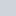# Exponential and Logarithmic Functions in PHP

Author — Nitish Kumar

## PHP `exp()` Function

### PHP `exp()` Usage

The PHP `exp()` function will give you `e` raised to the power of `arg`.

### PHP `exp()` Syntax

`` exp ( float \$arg ) : float``

### PHP `exp()` Parameters

1. `arg` — The argument to process

### PHP `exp()` Return Value

The PHP `exp()` function returns ‘e’ raised to the power of `arg`

### PHP `exp()` Working Examples

#### 1. exp() example

``````<?php
echo exp(12) . "\n";
echo exp(5.7);
?>``````

Output of the above code:

``````1.6275E+005
298.87``````

### Important Points about PHP `exp()` Function

1. `e`‘ is the base of the natural system of logarithms, or approximately 2.718282.

## PHP `expm1()` Function

### PHP `expm1()` Usage

The PHP `expm1()` function will give you exp(number) – 1, computed in a way that is accurate even when the value of number is close to zero .

### PHP `expm1()` Syntax

`` expm1 ( float \$arg ) : float``

### PHP `expm1()` Parameters

1. `arg` — The argument to process

### PHP `expm1()` Return Value

The PHP `expm1()` function returns ‘e’ to the power of `arg` minus one

### Changelog for PHP expm1() Function

5.3.0 — This function is now available on all platforms

## PHP `log10()` Function

### PHP `log10()` Usage

The PHP `log10()` function will give you the base-10 logarithm of `arg`.

### PHP `log10()` Syntax

`` log10 ( float \$arg ) : float``

### PHP `log10()` Parameters

1. `arg` — The argument to process

### PHP `log10()` Return Value

The PHP `log10()` function returns the base-10 logarithm of `arg`

## PHP `log1p()` Function

### PHP `log1p()` Usage

The PHP `log1p()` function will give you log(1 + number), computed in a way that is accurate even when the value of number is close to zero .

### PHP `log1p()` Syntax

`` log1p ( float \$number ) : float``

### PHP `log1p()` Parameters

1. `number` — The argument to process

### PHP `log1p()` Return Value

The PHP `log1p()` function returns log(1 + `number`)

### Changelog for PHP log1p() Function

5.3.0 — This function is now available on all platforms

## PHP `log()` Function

### PHP `log()` Usage

The PHP `log()` function will give you logbase `arg`, otherwise `log()` return the natural logarithm of `arg`.

### PHP `log()` Syntax

`` log ( float \$arg [, float \$base = M_E ] ) : float``

### PHP `log()` Parameters

1. `arg` — The value to calculate the logarithm for

2. `base` — The optional logarithmic base to use (defaults to ‘e’ and so to the natural logarithm).

### PHP `log()` Return Value

The PHP `log()` function returns the logarithm of `arg` to `base`, if given, or the natural logarithm.

## PHP `pow()` Function

### PHP `pow()` Usage

The PHP `pow()` function will give you `base` raised to the power of `exp`.

### PHP `pow()` Syntax

`` pow ( number \$base , number \$exp ) : number``

### PHP `pow()` Parameters

1. `base` — The base to use

2. `exp` — The exponent

### PHP `pow()` Return Value

The PHP `pow()` function returns d with integer type, otherwise it will be returned as a float.

### PHP `pow()` Working Examples

#### 1. Some examples of pow()

``````<?php
var_dump(pow(2, 8)); // int(256)
echo pow(-1, 20); // 1
echo pow(0, 0); // 1
echo pow(10, -1); // 0.1
echo pow(-1, 5.5); // PHP >=5.2.2: NAN
echo pow(-1, 5.5); // PHP <5.2.2: -NAN
?>``````

### Important Points about PHP `pow()` Function

1. In PHP 5.6 onwards, you may prefer to use the ** operator.

2. This function will convert all input to a number, even non-scalar values, which could lead to weird results.

### Additional Tips from Fellow Developers

Contributed By: chris

``````Many notations use "^" as a power operator, but in PHP (and other C-based languages) that is actually the XOR operator. You need to use this 'pow' function, there is no power operator.
i.e. 3^2 means "3 XOR 2" not "3 squared".
It is particular confusing as when doing Pythagoras theorem in a 'closet points' algorithm using "^" you get results that look vaguely correct but with an error.``````

Contributed By: gilthans

``Note that pow(0, 0) equals to 1 although mathematically this is undefined.``

## PHP `sqrt()` Function

### PHP `sqrt()` Usage

The PHP `sqrt()` function will give you the square root of `arg`.

### PHP `sqrt()` Syntax

`` sqrt ( float \$arg ) : float``

### PHP `sqrt()` Parameters

1. `arg` — The argument to process

### PHP `sqrt()` Return Value

The PHP `sqrt()` function returns the square root of `arg` or the special value NAN for negative numbers.

### PHP `sqrt()` Working Examples

#### 1. sqrt() example

``````<?php
// Precision depends on your precision directive
echo sqrt(9); // 3
echo sqrt(10); // 3.16227766 ...
?>``````

Rate this post —(No Ratings Yet)Loading...

Tags: |

Subscribe
Notify of